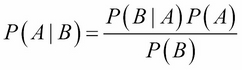•#### Big Data Analytics with Java#### Overview of this book

This book covers case studies such as sentiment analysis on a tweet dataset, recommendations on a movielens dataset, customer segmentation on an ecommerce dataset, and graph analysis on actual flights dataset. This book is an end-to-end guide to implement analytics on big data with Java. Java is the de facto language for major big data environments, including Hadoop. This book will teach you how to perform analytics on big data with production-friendly Java. This book basically divided into two sections. The first part is an introduction that will help the readers get acquainted with big data environments, whereas the second part will contain a hardcore discussion on all the concepts in analytics on big data. It will take you from data analysis and data visualization to the core concepts and advantages of machine learning, real-life usage of regression and classification using Naïve Bayes, a deep discussion on the concepts of clustering,and a review of simple neural networks on big data using deepLearning4j or plain Java Spark code. This book is a must-have book for Java developers who want to start learning big data analytics and want to use it in the real world.
Big Data Analytics with JavaCreditswww.PacktPub.comCustomer FeedbackPrefaceFree Chapter
Big Data Analytics with JavaFirst Steps in Data AnalysisData VisualizationBasics of Machine LearningRegression on Big DataNaive Bayes and Sentiment AnalysisDecision TreesEnsembling on Big DataRecommendation SystemsClustering and Customer Segmentation on Big DataMassive Graphs on Big DataReal-Time Analytics on Big DataDeep Learning Using Big DataIndex## Bayes theorem

The Bayes theorem is based on the concept of learning from experience, that is, using a sequence of steps to come to a prediction. It is the calculation of probability based on prior knowledge of occurrences that might have led to the event. Bayes theorem is given by the following formula:Where:

Probability Value

Description

P(A | B)

Conditional probability of event A given that event B has occurred.

P(B | A)

Conditional probability of event B given that event A has occurred.

P(A)

Individual probability of event A without regard to event B.

P(B)

Individual probability of event B without regard to event A.

Let's understand this using the same example as we used previously. Suppose we picked one green triangle randomly from a set then what is the probability that it came from Set-1?

Before we run the bayes theorem formula we will first calculate the individual probabilities:

• Probability of randomly picking a set from one of the two sets, Set-1 and Set-2

Since there...